# Perpendicular Lines – Definition, Symbol, Properties, Examples

## What are Perpendicular Lines?

Two distinct lines intersecting each other at $90^{\circ}$ or at a right angle are called perpendicular lines.

Example:

Here, AB is perpendicular to XY because AB and XY intersect each other at $90^{\circ}$.

Non-Example:

## Definition of Perpendicular Lines

In geometry, perpendicular lines are defined as two lines that meet or intersect each other at right angles $(90^{\circ})$. The term ‘perpendicular’ originated from the Latin word ‘perpendicularis,’ meaning a plumb line.

If two lines AB and CD are perpendicular, then we can write them as AB $\bot$ CD. The symbol $\bot$ is used to indicate the lines are perpendicular.

## Perpendicular Lines Around Us

We can observe many perpendicular lines in real life.

Some examples are: the sides of a set square, the arms of a clock, the corners of the blackboard, window and the Red Cross symbol.

Here are a few more examples!

## Perpendicular Symbol

Perpendicular lines are represented by the symbol, ‘$\bot$’. Suppose, m and n are two lines intersecting each other at 90 degrees, then they are perpendicular to each other and are represented as m $\bot$ n. The point of intersection of perpendicular lines is called the foot of the perpendicular.

## Properties of perpendicular lines

• These lines always intersect at right angles.
• If two lines are perpendicular to the same line, they are parallel to each other and will never intersect.  For example k and l are two lines and both are perpendicular to the same line r, then lines k and l are parallel to each other
• Adjacent sides of a square and a rectangle are always perpendicular to each other.

## Construction Of Perpendicular Lines

We can draw perpendicular lines for a given line in two ways.

• Using a protractor
• Using a compass

### Drawing A Perpendicular Line Using Protractor

Step 1: Let m be the given line and A the given point on it.

Step 2: Place the protractor on the line m such that its base line coincides with m, and its centre falls on A.

Step 3: Mark a point B against the 90 degree mark on the protractor.

Step 4: Draw a line n passing through A and B.

Then line m $bot$ line n at A.

### Drawing A Perpendicular Line At A Given Point On A Line Using Compass

Step 1: Place a compass tip on the given point A. Take an appropriate radius and draw an arc on either side of the given point.

Step 2: Stretch the compass wider. Place the compass tip on the new points and make a small arc above the given line. The two new arcs will intersect.

Step 3: Use the ruler to join the given point A and the point B where the arcs intersect.

## Conclusion

Give your children opportunities to observe perpendicular lines in objects or places around them, such as a tall tree on the ground, an electric pole on the pavement, railway intersection, corner of two adjacent walls, and high buildings.

Let’s solve a few examples for better understanding.

## Solved Examples On Perpendicular Lines

Example 1: State whether a given set of lines are perpendicular or parallel.

Solution:

1. Parallel lines
2. Perpendicular lines
3. Perpendicular lines
4. Parallel lines

Example 2: Write a relation between the line segments indicated by the arrow in each shape.

Solution:

1. AB $\bot$ AE
2.  PQ $\left|\right|$ SR
3. PQ $\left|\right|$ NM
4. AB $\bot$ AD

Example 3: The diagonals of rhombus are perpendicular to each other. How many right angles are formed at the intersection?

Solution:

Since the lines AC and BD intersect each other at 90 degrees, there are 4 right angles at the intersecting point.

$\angle \text{AED} = \angle \text{DEC} = \angle \text{CEB} = \angle \text{BEA} = 90$ degrees

Example 4: Name two dimensional shapes having perpendicular lines.

Solution:

Example 5: If AB $\left|\right|$ CD and CD $\left|\right|$ EF. What can you say about AB and EF?

Solution:

Line AB is perpendicular to line CD.

Also, line CD is perpendicular to line EF.

When two lines are perpendicular to the same line, then the 2 lines are parallel to each other.

Thus, lines AB and EF are parallel to each other.

Example 6: Are these lines perpendicular?

Solution:

No, these lines are not perpendicular because they do not intersect at right angles.

Example 7: Are these lines perpendicular?

Solution: Yes, these are perpendicular lines. They intersect at $90^{\circ}$.

## Practice Problems On Perpendicular Lines

1

### Identify the pair of perpendicular line segments in a given shape.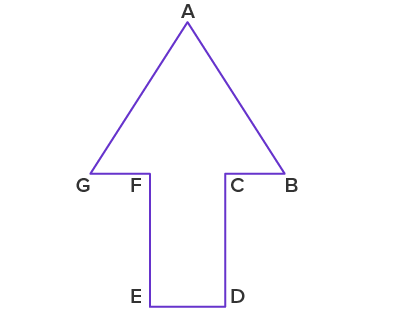AG and AB
FE and ED
FE and CD
CB and AB
CorrectIncorrect
Correct answer is: FE and ED
FE and ED are perpendicular line segments.
2

### Each object has a highlighted pair of lines. Which of this object highlighted pair of lines does not show perpendicular lines?

ABC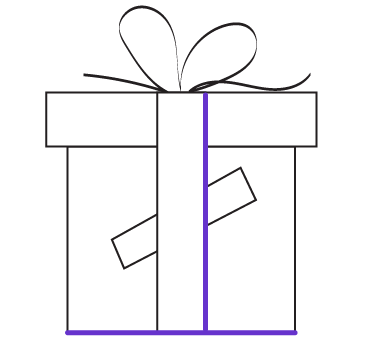D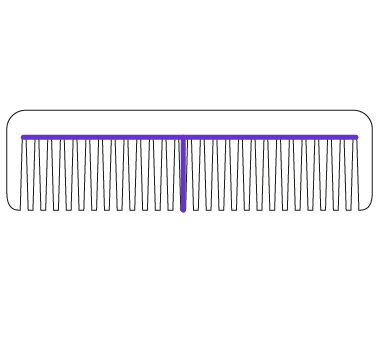CorrectIncorrect
Candle. Its highlighted pair of lines shows parallel lines.
3

### At what time the hands of the clock will be perpendicular to each other?

12 o’clock
6 o’clock
3 o’clock
5 o’clock
CorrectIncorrect
At 3 o’clock the hands of the clock are at 90 degrees to each other. So they are perpendicular to each other.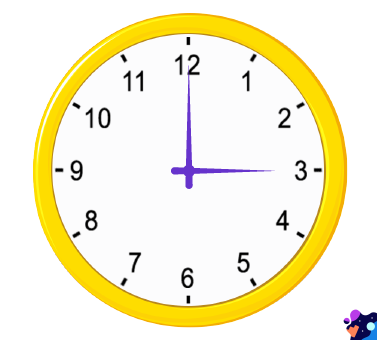4

### How many lines are perpendicular to line CD in a given figure?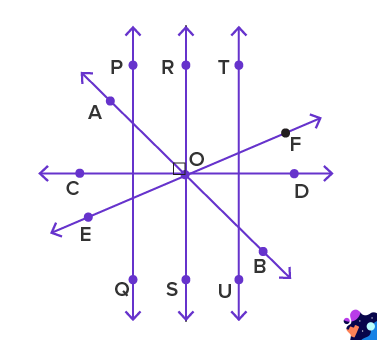1
2
3
4
CorrectIncorrect
Line PQ, line RS and line TU are perpendicular to CD. So, there are 3 lines perpendicular to line CD in the given figure.
5

### At what time will the hands of the clock be perpendicular?

03:40 pm
04:00 pm
09:00 am
10:10 am
CorrectIncorrect
The hands of the clock intersect at right angles when the time is 9:00 am.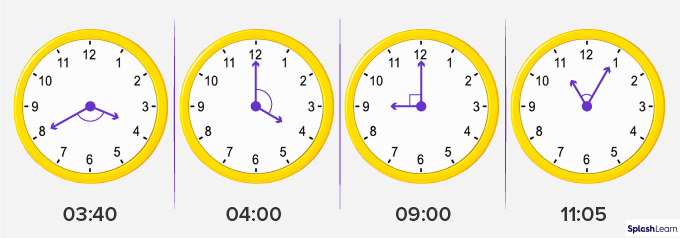6

### Perpendicular lines intersect each other at _____ angle.

Right
Obtuse
Acute
Straight
CorrectIncorrect
Perpendicular lines intersect each other at $90^{\circ}$. $90^{\circ}$ angles are known as right angles.
7

### In which of the following objects can we find perpendicular lines?

Ball
Birthday hat
Banana
Laptop
CorrectIncorrect
Laptop has edges intersecting at right angles. All other images do not have lines intersecting at right angles.

## Frequently Asked Questions On Perpendicular Lines

We can define a perpendicular line as two straight lines joined to a common point making a $90^{\circ}$ angle with each other. Perpendicular lines are those lines that intersect each other at 90 degrees.

These lines always intersect at right angles.

The perpendicular symbol is $\left|\right|$. It is used between the two lines to show that they are perpendicular to each other.

Perpendicular lines intersect at right angles (90 degree) while parallel lines are parallel to each other and never intersect. Graphically, parallel lines are two or more straight lines that do not touch each other even after extending them. However, perpendicular lines intersect each other at $90^{\circ}$ .

No, not all intersecting lines are perpendicular to each other. They may intersect at different angles other than 90 degrees.

Parallel lines never touch each other while perpendicular lines meet each other at the intersecting point. Thus, you cannot have two lines that are both parallel and perpendicular to each other.

A perpendicular line must be at $90^{\circ}$ with the other line. You can draw only one perpendicular to a given line that passes through the given point.

A perpendicular line must be at $90^{\circ}$ with the other line. You can draw one perpendicular to a given line passing through a given point.  As you can draw many lines in this manner, there can be an infinite number of perpendicular lines on a given line.